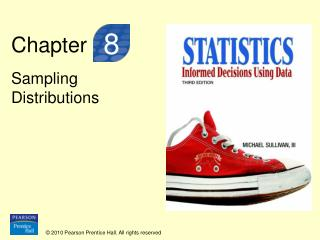Download PresentationChapter

# Chapter - PowerPoint PPT PresentationDownload Presentation## Chapter

- - - - - - - - - - - - - - - - - - - - - - - - - - - E N D - - - - - - - - - - - - - - - - - - - - - - - - - - -
##### Presentation Transcript

1. Chapter 8 Sampling Distributions

2. Section 8.2 Distribution of the Sample Proportion

3. Objectives • Describe the sampling distribution of a sample proportion • Compute probabilities of a sample proportion

4. Objective 1 • Describe the Sampling Distribution of a Sample Proportion

5. Point Estimate of a Population Proportion Suppose that a random sample of size n is obtained from a population in which each individual either does or does not have a certain characteristic. The sample proportion, denoted (read “p-hat”) is given by where x is the number of individuals in the sample with the specified characteristic. The sample proportion is a statistic that estimates the population proportion, p.

6. Parallel Example 1: Computing a Sample Proportion In a Quinnipiac University Poll conducted in May of 2008, 1,745 registered voters nationwide were asked whether they approved of the way George W. Bush is handling the economy. 349 responded “yes”. Obtain a point estimate for the proportion of registered voters who approve of the way George W. Bush is handling the economy.

7. Parallel Example 1: Computing a Sample Proportion In a Quinnipiac University Poll conducted in May of 2008, 1,745 registered voters nationwide were asked whether they approved of the way George W. Bush is handling the economy. 349 responded “yes”. Obtain a point estimate for the proportion of registered voters who approve of the way George W. Bush is handling the economy. Solution:

8. Parallel Example 2: Using Simulation to Describe the Distribution of the Sample Proportion According to a Time poll conducted in June of 2008, 42% of registered voters believed that gay and lesbian couples should be allowed to marry. Describe the sampling distribution of the sample proportion for samples of size n=10, 50, 100.

9. Key Points from Example 2 • Shape: As the size of the sample, n, increases, the shape of the sampling distribution of the sample proportion becomes approximately normal. • Center: The mean of the sampling distribution of the sample proportion equals the population proportion, p. • Spread:The standard deviation of the sampling distribution of the sample proportion decreases as the sample size, n, increases.

10. Sampling Distribution of For a simple random sample of size n with population proportion p: • The shape of the sampling distribution of is approximately normal provided np(1-p)≥10. • The mean of the sampling distribution of is . • The standard deviation of the sampling distribution of is

11. Sampling Distribution of • The model on the previous slide requires that the sampled values are independent. When sampling from finite populations, this assumption is verified by checking that the sample size n is no more than 5% of the population size N (n ≤ 0.05N). • Regardless of whether np(1-p) ≥10 or not, the mean of the sampling distribution of is p, and the standard deviation is

12. Parallel Example 3: Describing the Sampling Distribution of the Sample Proportion According to a Time poll conducted in June of 2008, 42% of registered voters believed that gay and lesbian couples should be allowed to marry. Suppose that we obtain a simple random sample of 50 voters and determine which believe that gay and lesbian couples should be allowed to marry. Describe the sampling distribution of the sample proportion for registered voters who believe that gay and lesbian couples should be allowed to marry.

13. Solution The sample of n=50 is smaller than 5% of the population size (all registered voters in the U.S.). Also, np(1-p)=50(0.42)(0.58)=12.18≥10. The sampling distribution of the sample proportion is therefore approximately normal with mean=0.42 and standard deviation= . (Note: this is very close to the standard deviation of 0.072 found using simulation in Example 2.)

14. Objective 2 • Compute Probabilities of a Sample Proportion

15. Parallel Example 4: Compute Probabilities of a Sample Proportion According to the Centers for Disease Control and Prevention, 18.8% of school-aged children, aged 6-11 years, were overweight in 2004. • In a random sample of 90 school-aged children, aged 6-11 years, what is the probability that at least 19% are overweight? • Suppose a random sample of 90 school-aged children, aged 6-11 years, results in 24 overweight children. What might you conclude?

16. Solution • n=90 is less than 5% of the population size • np(1-p)=90(.188)(1-.188)≈13.7≥10 • is approximately normal with mean=0.188 and standard deviation = • In a random sample of 90 school-aged children, aged 6-11 years, what is the probability that at least 19% are overweight? , P(Z>0.05)=1-0.5199=0.4801

17. Solution • is approximately normal with mean=0.188 and standard deviation = 0.0412 • Suppose a random sample of 90 school-aged children, aged 6-11 years, results in 24 overweight children. What might you conclude? , P(Z>1.91)=1-0.9719=0.028. We would only expect to see about 3 samples in 100 resulting in a sample proportion of 0.2667 or more. This is an unusual sample if the true population proportion is 0.188.